Navigation Panel:(These buttons explained below)Question Corner and Discussion Area

# Tetrahedral and 4-Tetrahedral Numbers

Asked by Warren Kendrick on November 4, 1997:
The tetrahedronal numbers: 1, 4, 10, 20, 35, . . . are derived by adding the triangular numbers: 1 + 3 + 6 + 10 + 15 + · · · + (n)(n+1)/2. At one time I derived a formula for the sum through the mth term of the tetrahedronal numbers, and also the sum of the numbers for a 4-tetrahedron: 1, 5, 15, 35, 70, . . . , which are of course the sum of the tetrahedronal numbers. (Tetrahedronal numbers are best described by placing larger and larger triangular layers of 3-spheres under the previous triangular layer of spheres, forming a tetrahedronal pyramid.) Can you provide these formulas? Thanks.
These numbers are all expressible as binomial coefficients. If we letdenote the nth triangular number,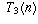the nth tetrahedral number, and so on, thenYou can prove this by induction noting that eachequals 1, that the formula is correct when k=2, and the difference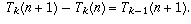You could also prove this by a more "brute-force" approach, using the formulas for the sums of powers:and so on. To arrive at these formulas you can again proceed by brute force (make the intelligent guess that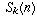should be a polynomial of degree n+1 and plug in enough values to solve for the coefficients), or else employ a trick like the following:

Write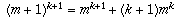plus terms involving lower powers of m. When you add up the LHS as m ranges from 0 to n, you get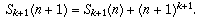When you add up the RHS, you get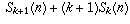plus terms involving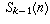down throughThis gives youThe termscancel, and you are left with a formula that expressesin terms ofdown through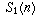.

For example, here is how to use the above technique to arrive at the formula for: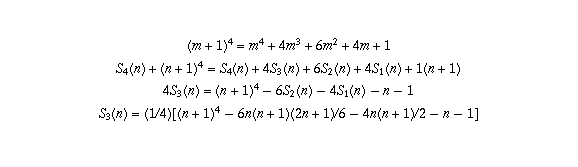and simplifying gives the above formula. (Note that the reason n+1 appears at the end of the second line is that we are adding up the first line as m ranges from 0 to n; that means the final constant term "1" is added up n+1 times.)

Now that you have formulas for S_k(n), it is easy to find formulas for the tetrahedral numbers. For example,[ Submit Your Own Question ] [ Create a Discussion Topic ]

This part of the site maintained by (No Current Maintainers)
Last updated: April 19, 1999
Original Web Site Creator / Mathematical Content Developer: Philip Spencer
Current Network Coordinator and Contact Person: Joel Chan - mathnet@math.toronto.edu

Navigation Panel: